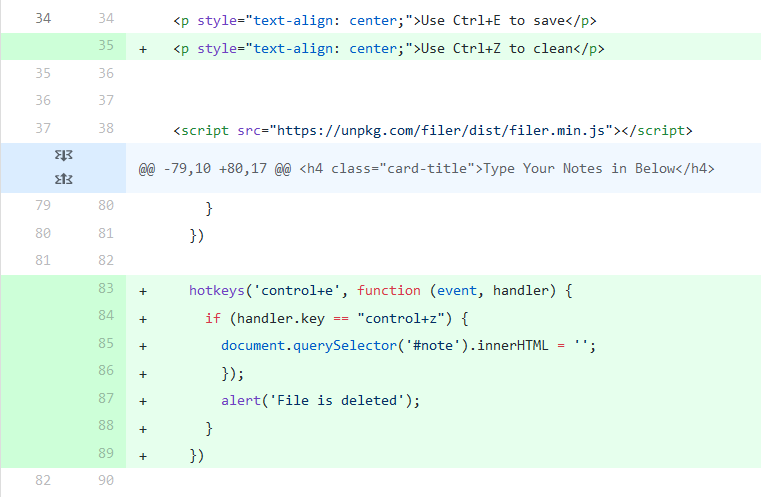## Posts

Showing posts from September, 2019

### Algorithm Selection Lab

Background This lab is to help understanding software optimize. As technique developing, the contents a software can be represented are rich. We use more high quality images, audios and videos. High quality means more space need, less battery usage. The mission is to find the balance between them.
Example: Basic Sound Program aarchie server vol.h

#define SAMPLES 5000000

vol1.c

// Function to scale a sound sample using a volume_factor
// in the range of 0.00 to 1.00.
static inline int16_t scale_sample(int16_t sample, float volume_factor) {
return (int16_t) (volume_factor * (float) sample);
}

int main() {

// Allocate memory for large in and out arrays
int16_t*        data;
data = (int16_t*) calloc(SAMPLES, sizeof(int16_t));

int             x;
int             ttl = 0;

// Seed the pseudo-random number generator
srand(1);

// Fill the array with random data
for (x = 0; x < SAMPLES; x++) {
data[x] = (rand()%…